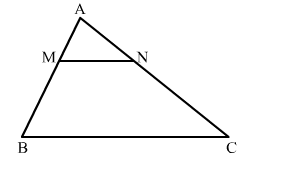# In the given figure, MN∥BC and AM : MB = 1 : 2.Question:

In the given figure, MN∥BC and AM : MB = 1 : 2.

Find $\frac{\operatorname{area}(\triangle \mathrm{AMN})}{\operatorname{area}(\triangle \mathrm{ABC})}$Solution:

We have
AM : MB = 1 : 2

$\Rightarrow \frac{\mathrm{MB}}{\mathrm{AM}}=\frac{2}{1}$

Adding 1 to both sides, we get

$\Rightarrow \frac{\mathrm{MB}}{\mathrm{AM}}+1=\frac{2}{1}+1$

$\Rightarrow \frac{\mathrm{MB}+\mathrm{AM}}{\mathrm{AM}}=\frac{2+1}{1}$

$\Rightarrow \frac{\mathrm{AB}}{\mathrm{AM}}=\frac{3}{1}$

Now, In △AMN and △ABC
∠AMN = ∠ABC         (Corresponding angles in MN∥BC)
∠ANM = ∠ACB         (Corresponding angles in MN∥BC)
By AA similarity criterion, △AMN ∼ △ABC
If two triangles are similar, then the ratio of their areas is equal to the ratio of the squares of their corresponding sides.

$\therefore \frac{\operatorname{area}(\triangle \mathrm{AMN})}{\operatorname{area}(\triangle \mathrm{ABC})}=\left(\frac{\mathrm{AM}}{\mathrm{AB}}\right)^{2}=\left(\frac{1}{3}\right)^{2}=\frac{1}{9}$